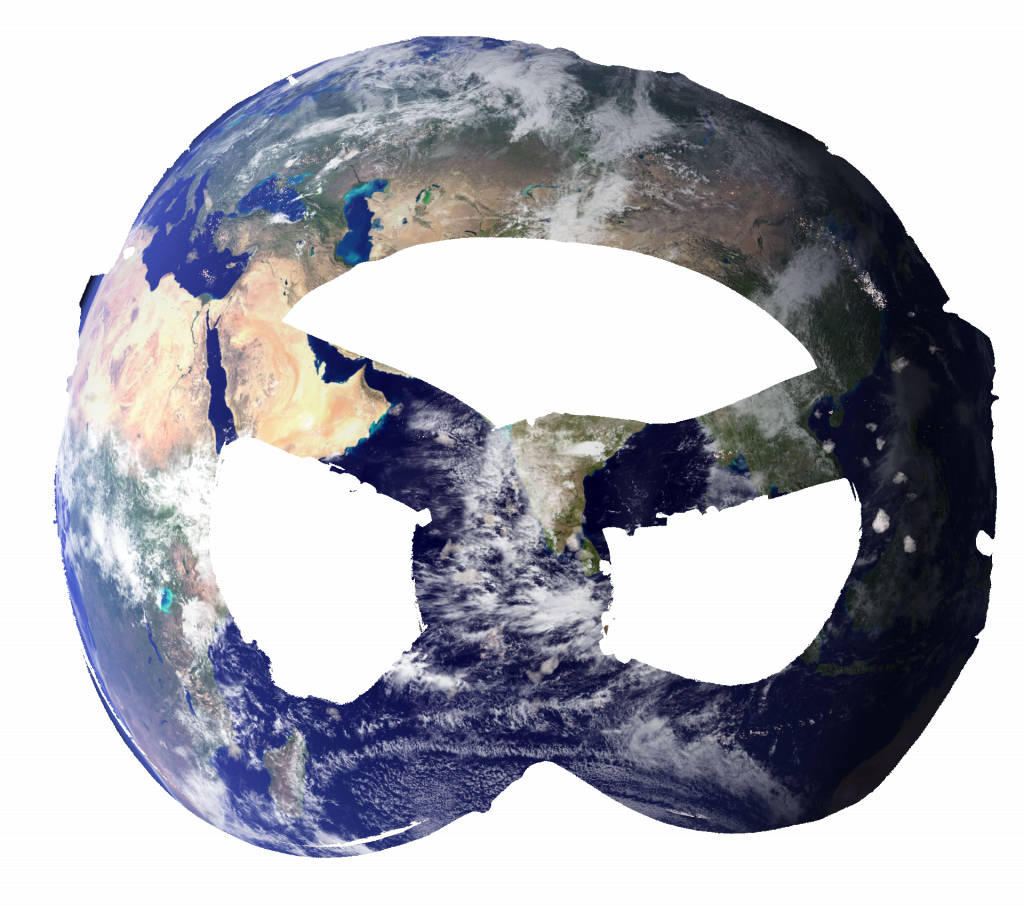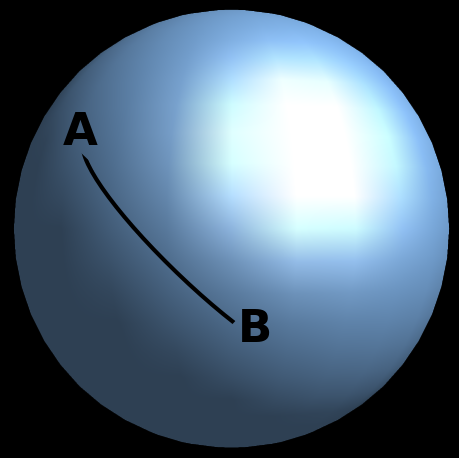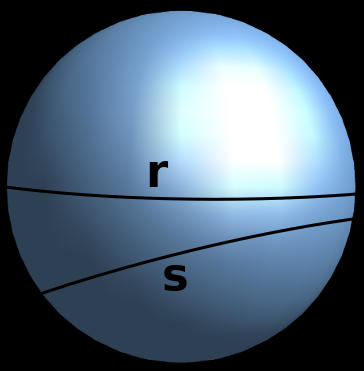# Earth-Prezel Hypothesis

The situation that we will present below serves as an example for the idea of hypothesis manipulation and its role in mathematics. For although some things seem “trivial” or “obvious” and their denial is “absurd” or “ridiculous”, when we assume these oddities, we can arrive at different ways of seeing the world.

Suppose you are on a plane when the pilot informs you that he does not have enough fuel to land on the flight path. Both “know” that the Earth is a globe, but given the circumstances the pilot asked the computer to analyze a flight path assuming that the Earth was a pretzel and saw that it would be possible to land the plane.

Both are fully convinced that the Earth is a globe, but they also know that this means the plane crashed… but what if they were wrong in that respect? They could survive this situation, even if the hypothesis of the Earth being a pretzel seems absurd to them.

For clarification, pretzel is a type of bread of German origin, which can be sweet or salty. It is shaped like a strange knot, and the planet in the shape of a pretzel would look something like the figure belowEuclid of Alexandria was a great Greek mathematician considered to be the father of geometry, he lived about 300 years before Christ and described geometry from 5 postulates (propositions considered trivial and which we must assume to be true without proving). His “obvious” way of describing geometry in the plane led to the development of Euclidean geometry, which proposes for example “the shortest distance between two points is a straight line”.

However, efforts have been made over the centuries against one of these postulates, known as the Postulate of Parallels and which can be stated as follows:

It is true that if a line when cutting two others forms internal angles on the same side, the sum of which is less than two right angles, then the two lines, if continued, will meet on the side where the angles whose sum is less than two right angles.

In a less complicated way for us who are already born breathing geometry, the postulate says that any two non-parallel lines will intersect.

Several mathematicians sought to deduce this postulate from the 4 other postulates and thus “prove” that geometry as proposed by Euclides, could be described only with 4 and not with 5 absolute truths. However, whenever they tried to “assume” that if this postulate were false it would lead to an absurd statement, they would arrive at something different, but not absurd, something that came to be studied as non-Euclidean geometries. Thus, although they seem “obvious”, the denial of a postulate led us to different forms of geometry that are not Euclidean (that is, the one that is based on the 5 postulates are true).

A simple way to exemplify non-Euclidean geometry is to imagine an ant on top of a plastic ball. The shortest distance between two points on the ball is certainly not a straight line (since the ant will not cross the interior of the object), but an arc of circumference with amplitude AB, which represents the path between two points of this ball. In this same universe of the plastic ball, any position that the ant wants to reach will be at most one arc of circumference with 180 degrees and radius equal to that of the ball. In this case, for the ant to reach the most distant point possible on the ball, there are infinite equally short paths.

Similarly, two lines on this ball would be represented by circumferences inscribed on its surface and which may never touch even if they are not parallel. Thus, with the simplistic situation of an ant in a plastic ball, we can observe contradictions that Euclidean geometry would have in extending this universe of non-Euclidean properties.With that, we emphasize the invitation to the reader to learn more about mathematics, but not through calculations and formulas (which are necessary to verify the hypotheses, but not enough to create them), but in this “game” of playing with the hypotheses. For even when everything seems “obvious”, mathematicians place themselves in the duty to assume that some things may be different and to see the consequences that these ideas provide for the analysis of the situation as a whole.